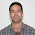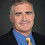## Tuesday, November 12, 2002

### The Union of Complexity Classes

We often see the intersection of two classes as an interesting class in and of itself. For example factoring is in NP∩co-NP. In some cases you get interesting equalities, like that ZPP is equal to RP∩co-RP. But we rarely see the union of two classes. Every wonder why?

In fact, no complexity class can be the nontrivial union of two other classes. To formalize and prove this statement we need some definitions.

Let A and B be subsets of {0,1}*. We define the join, A⊕B, as the union of {0x | x is in A} and {1y | y is in B}. Given a set C we define the 0-projection of C as {x | 0x is in C} and the 1-projection of C as {y | 1y is in C}. Note that the 0-projection of A⊕B is just A and the 1-projection is just B.

Essentially every complexity class is closed under joins and projections. For example if A and B are in NP then A⊕B is also in NP. The fact that no complexity class is the nontrivial union of other classes follows from the following Lemma.

Lemma: Let E, F and G be classes of languages that are closed under joins and projections and G = EF. Then either G = E or G = F.

Proof: Suppose the lemma is false. Let A be a set in G-E and B be a set in G-F. Let C = A⊕B. We have that C is in G since G is closed under joins. Thus C is in either E or F. Suppose C is in E. Since E is closed under projections, we have A is in E a contradiction. If C is in F then B is in F also a contradiction.

1.Hi!! Lance, I suspect that every subset of a language in NP is in NP too. How right or wrong am I? Indeed, I'm wondering if each subset of a language in P is also in P, because maybe it might depend whether P is equal to NP or not. I would appreciate your answer very much, please.

1.Sigma^* (the set of all strings) is in both P and NP and every set (including the halting problem) is a subset of Sigma^*. So it is not the case that every subset of a language in P or NP is in P or NP.

2.Thanks Lance. What a pity!!! Certainly the following proof would be revelant,

http://hal.archives-ouvertes.fr/hal-01070568

if my suspicious were right. Unfortunately, I'm not. However, I would like to share it, maybe you or somebody else see some benefits on this proof. Here is the abtract,

<<$ONE-IN-THREE \ 3SAT$ is the problem of deciding whether a given boolean formula $\phi$ in $3CNF$ has a truth assignment such that each clause in $\phi$ has exactly one true literal. This problem is $NP-complete$. We define a similar language: $ZERO-ONE-IN-THREE \ 3SAT$ is the problem of deciding whether a given boolean formula $\phi$ in $3CNF$ has a truth assignment such that each clause in $\phi$ has at most one true literal. Indeed, $ONE-IN-THREE \ 3SAT \subseteq ZERO-ONE-IN-THREE \ 3SAT$. In this work, we prove $ZERO-ONE-IN-THREE \ 3SAT \in P$.>>

I hope it might be interesting even though is a very trivial proof.

2.Professor in a book of Computational Complexity there is a question that states: If L1 is NP-complete and L2 is co-NP-complete, then the intersection of L1 with L2 is DP-complete (True or false?). I suspect the answer is true. However, I'm not quite sure. Could you help me to clarify this doubt, please? Thanks in advance.

1.I won't answer questions in textbooks. But here's a hint: Intersections of sets from complexity classes can do funny things.

3.Hi Lance!!! I don't know whether LOGSPACE is closed under union or not. I have google it, but I haven't found anything yet. I would appreciate your answer very much, please.

1.Yes, the usual algorithm to compute the union of two log space sets will still only use logarithmic space.

2.I guess this can be apply not only for two sets, but for the union of infinite log space sets too. Am I wrong?

4.Thank you so much...